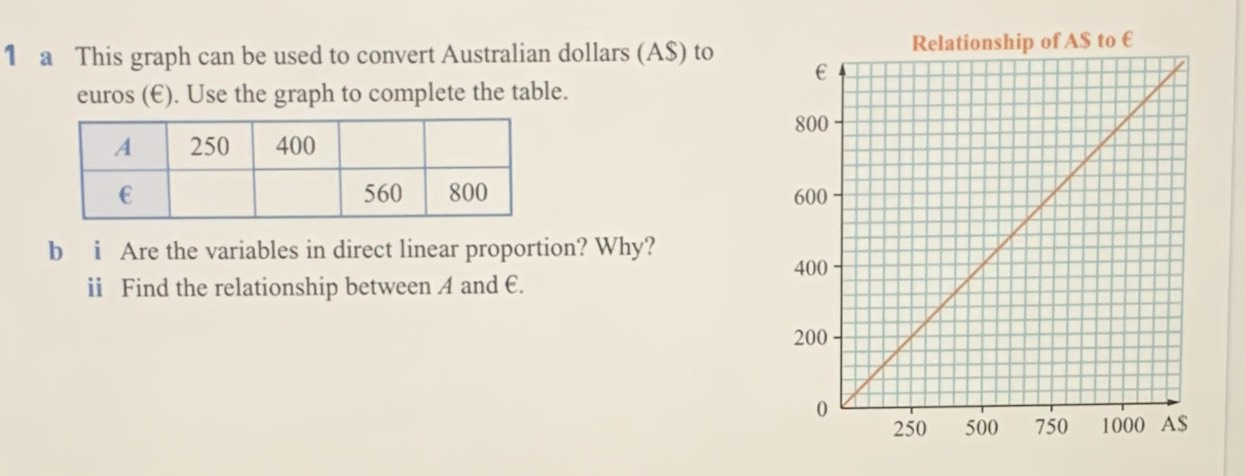### ¿Todavía tienes preguntas de matemáticas?

Pregunte a nuestros tutores expertos
Algebra
Pregunta$$1$$ a This graph can be used to convert Australian dollars (AS) to euros $$( € )$$ . Use the graph to complete the table.

b i Are the variables in direct linear proportion? Why?

ii Find the relationship between $$A$$ and $$€ .$$

$$700$$         $$1000$$
$$200$$           $$320$$